`前言：` Hello大家好，我是Dream。 OpenCV是一个开源的计算机视觉库，可以用于实现各种图像和视频处理任务，包括红绿灯识别。可以帮助自动驾驶汽车、智能交通系统等设备准确地识别红绿灯的状态，以便做出正确的决策。今天，就有Dream带领大家复盘一下计算机视觉中最经典的实验：OpenCV进行红绿灯识别，一起来看看吧~

## 一、背景介绍

RGB颜色空间以R(Red:红）、G(Green:绿色)、 B(Blue:蓝）三种基本色为基础，进行不同程度的叠加，产生丰富而广泛的颜色，所以俗称三基色模式。在大自然中有无穷多种不同的颜色，而人眼只能分辨有限种不同的颜色，RGB棋式可表示一千六百多万种不同的颜色，在人跟看起来它非常接近大自然的颜色，故又称为自然色彩模式。红绿蓝代表可见光谱中的三种基木颜色或称为三原色，每一种颜色按其亮度的不同分为256个等级。当色光三原色重叠时，由于不同的混色比例能产生各种中间色。

RGB颜色空间最大的优点就是直观，容易理解。缺点是R、G、B这三个分量是高度相关的，即如果一个颜色的某一个分量发生了一定程度的改变，那么这个相色很可能要发生改变；人眼对于常见的 红绿蓝三色的敏感程度是不一样的，因此RGB颜色空间的均匀性非常差，且两种颜色之间的知觉差异色差不能表示为改颜色空间中两点间的距离，但是利用线性或非线性变换，则可以从RGB颜色空间推导出其他的颜色特征空间。

## 二、数据集介绍

`723`张红色交通灯图片：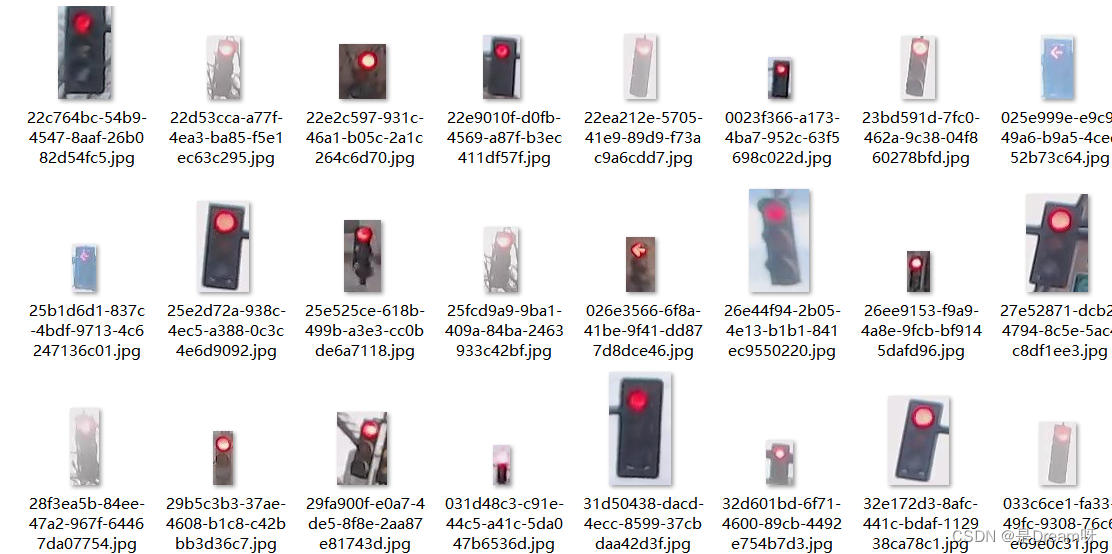`429`张绿色交通灯图片：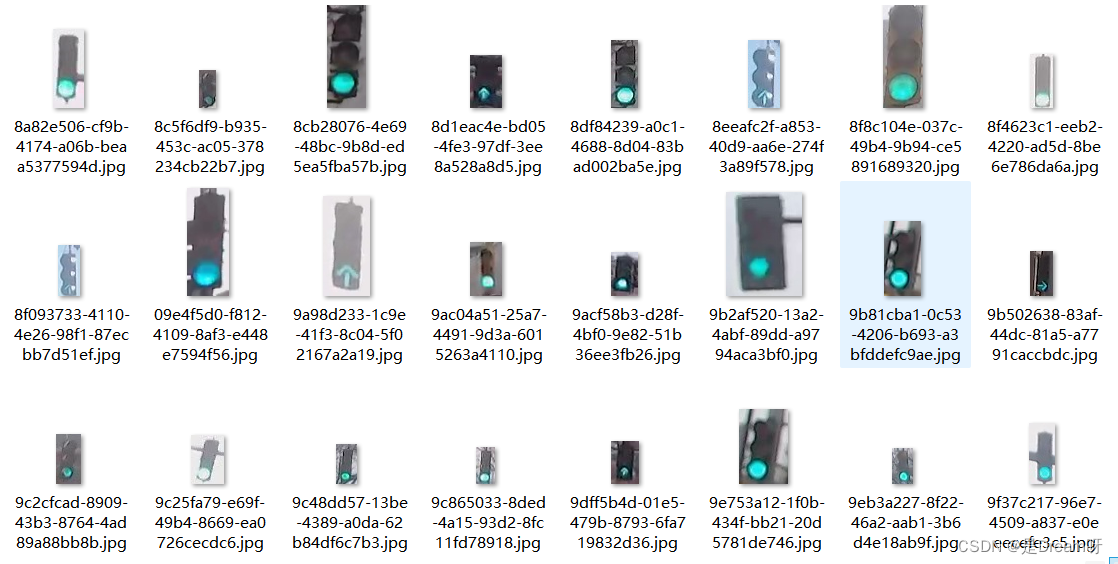`35`张黄色交通灯图片：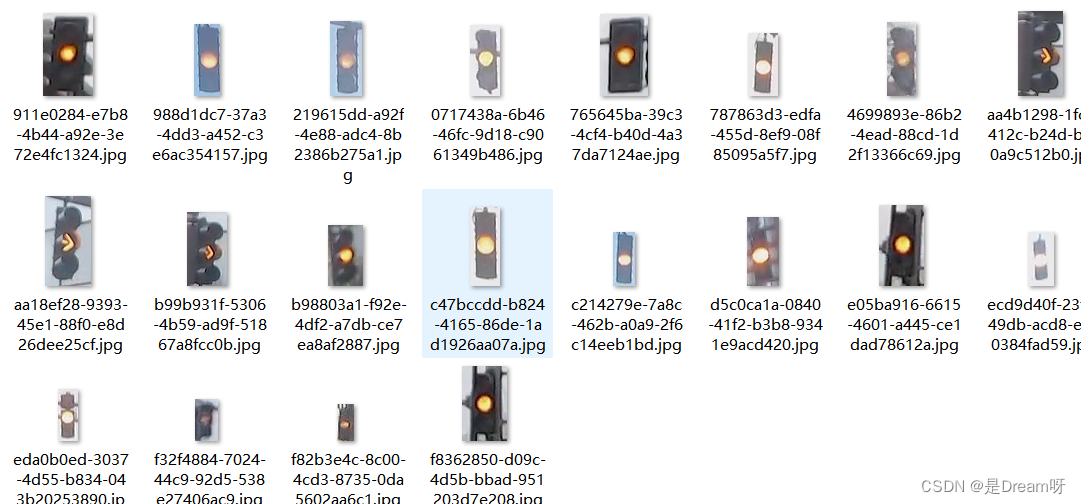## 三、加载数据集

### 1.导入库

``````# import some libs
import cv2
import os
import glob
import random
import numpy as np
import matplotlib.pyplot as plt
import matplotlib.image as mpimg
%matplotlib inline
``````

### 2.图像数据目录

``````IMAGE_DIR_TRAINING = "traffic_light_images/training/"
IMAGE_DIR_TEST = "traffic_light_images/test/"
``````

### 3.加载数据集

``````def load_dataset(image_dir):
'''
This function loads in images and their labels and places them in a list
image_dir:directions where images stored
'''
im_list =[]
image_types= ['red','yellow','green']

#Iterate through each color folder
for im_type in image_types:
file_lists = glob.glob(os.path.join(image_dir,im_type,'*'))
print(len(file_lists))
for file in file_lists:

if not im is None:
im_list.append((im,im_type))
return im_list
``````

## 四、数据可视化

• 对于第一个子图，使用`ax.imshow()`函数显示红灯图像，使用`ax.annotate()`函数添加标签信息，使用`ax.axis('off')`函数关闭坐标轴，使用`ax.set_title()`函数设置标题，最后使用`plt.show()`函数显示图像。

• 对于第二个子图和第三个子图，分别使用类似的方式显示黄灯图像和绿灯图像，并添加对应的标签信息和标题。

• 最后，使用`plt.show()`函数显示整个图像。

``````_,ax = plt.subplots(1,3,figsize=(5,2))
#red
img_red = IMAGE_LIST
ax.imshow(img_red)
ax.annotate(IMAGE_LIST,xy=(2,5),color='blue',fontsize='10')
ax.axis('off')
ax.set_title(img_red.shape,fontsize=10)
#yellow
img_yellow = IMAGE_LIST
ax.imshow(img_yellow)
ax.annotate(IMAGE_LIST,xy=(2,5),color='blue',fontsize='10')
ax.axis('off')
ax.set_title(img_yellow.shape,fontsize=10)
#green
img_green = IMAGE_LIST
ax.imshow(img_green)
ax.annotate(IMAGE_LIST,xy=(2,5),color='blue',fontsize='10')
ax.axis('off')
ax.set_title(img_green.shape,fontsize=10)
plt.show()
``````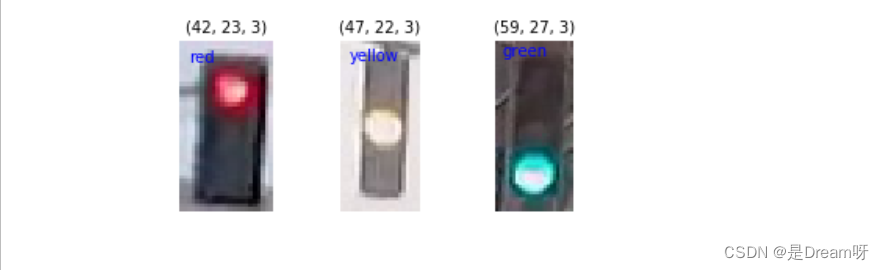## 五、数据预处理

### 1.输入

``````# 标准化输入图像，这里我们resize图片大小为32x32x3,这里我们也可以对图像进行裁剪、平移、旋转
def standardize(image_list):
'''
This function takes a rgb image as input and return a standardized version
image_list: image and label
'''
standard_list = []
#Iterate through all the image-label pairs
for item in image_list:
image = item
label = item
# Standardize the input
standardized_im = standardize_input(image)
# Standardize the output(one hot)
one_hot_label = one_hot_encode(label)
# Append the image , and it's one hot encoded label to the full ,processed list of image data
standard_list.append((standardized_im,one_hot_label))
return standard_list

def standardize_input(image):
#Resize all images to be 32x32x3
standard_im = cv2.resize(image,(32,32))
return standard_im
``````

### 2.输出

``````def one_hot_encode(label):
#return the correct encoded label.
'''
# one_hot_encode("red") should return: [1, 0, 0]
# one_hot_encode("yellow") should return: [0, 1, 0]
# one_hot_encode("green") should return: [0, 0, 1]
'''
if label=='red':
return [1,0,0]
elif label=='yellow':
return [0,1,0]
else:
return [0,0,1]
``````

## 六、特征提取

### 1.RGB to HSV

``````#Visualize
image_num = 0
test_im = Standardized_Train_List[image_num]
test_label = Standardized_Train_List[image_num]
#convert to hsv
hsv = cv2.cvtColor(test_im, cv2.COLOR_RGB2HSV)
# Print image label
print('Label [red, yellow, green]: ' + str(test_label))
h = hsv[:,:,0]
s = hsv[:,:,1]
v = hsv[:,:,2]
# Plot the original image and the three channels
_, ax = plt.subplots(1, 4, figsize=(20,10))
ax.set_title('Standardized image')
ax.imshow(test_im)
ax.set_title('H channel')
ax.imshow(h, cmap='gray')
ax.set_title('S channel')
ax.imshow(s, cmap='gray')
ax.set_title('V channel')
ax.imshow(v, cmap='gray')
``````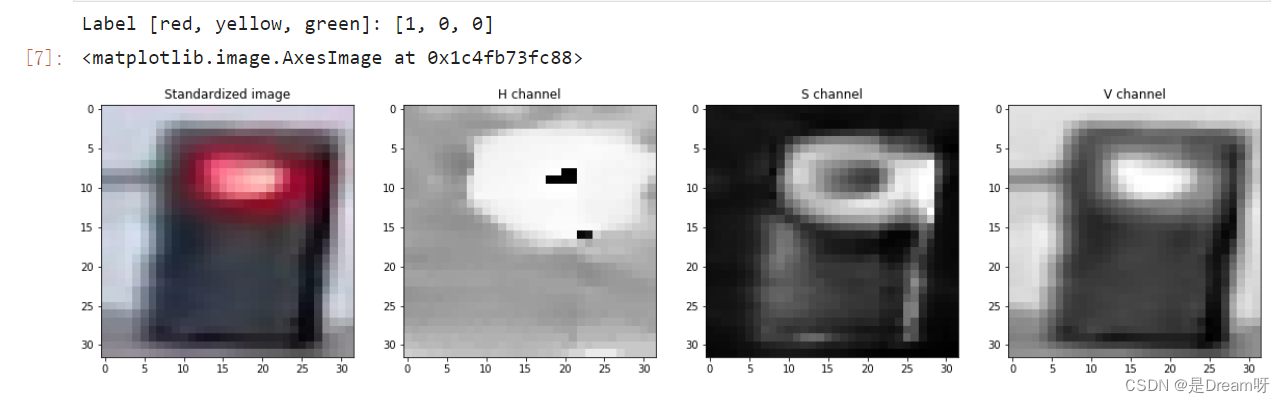HSV即色相、饱和度、明度（英语：Hue, Saturation, Value），又称HSB：

### 2.创建图像特征

``````def create_feature(rgb_image):
'''
Basic brightness feature
rgb_image : a rgb_image
'''
hsv = cv2.cvtColor(rgb_image,cv2.COLOR_RGB2HSV)

sum_brightness = np.sum(hsv[:,:,2])
area = 32*32
avg_brightness = sum_brightness / area#Find the average
return avg_brightness
``````

``````def high_saturation_pixels(rgb_image,threshold=80):
'''
Returns average red and green content from high saturation pixels
Usually, the traffic light contained the highest saturation pixels in the image.
The threshold was experimentally determined to be 80
'''
high_sat_pixels = []
hsv = cv2.cvtColor(rgb,cv2.COLOR_RGB2HSV)
for i in range(32):
for j in range(32):
if hsv[i][j] > threshold:
high_sat_pixels.append(rgb_image[i][j])
if not high_sat_pixels:
return highest_sat_pixel(rgb_image)

sum_red = 0
sum_green = 0
for pixel in high_sat_pixels:
sum_red+=pixel
sum_green+=pixel

avg_red = sum_red / len(high_sat_pixels)
avg_green = sum_green / len(high_sat_pixels)*0.8
return avg_red,avg_green
def highest_sat_pixel(rgb_image):
'''
Finds the highest saturation pixels, and checks if it has a higher green
or a higher red content
'''
hsv = cv2.cvtColor(rgb_image,cv2.COLOR_RGB2HSV)
s = hsv[:,:,1]

x,y = (np.unravel_index(np.argmax(s),s.shape))
if rgb_image[x,y,0] > rgb_image[x,y,1]*0.9:
return 1,0 #red has a higher content
return 0,1
``````

## 七、测试数据集

``````def estimate_label(rgb_image,display=False):
'''
rgb_image:Standardized RGB image
'''
return red_green_yellow(rgb_image,display)
def findNoneZero(rgb_image):
rows,cols,_ = rgb_image.shape
counter = 0
for row in range(rows):
for col in range(cols):
pixels = rgb_image[row,col]
if sum(pixels)!=0:
counter = counter+1
return counter
def red_green_yellow(rgb_image,display):
hsv = cv2.cvtColor(rgb_image,cv2.COLOR_RGB2HSV)
sum_saturation = np.sum(hsv[:,:,1])# Sum the brightness values
area = 32*32
avg_saturation = sum_saturation / area #find average

sat_low = int(avg_saturation*1.3)#均值的1.3倍，工程经验
val_low = 140
#Green
lower_green = np.array([70,sat_low,val_low])
upper_green = np.array([100,255,255])
#Yellow
lower_yellow = np.array([10,sat_low,val_low])
upper_yellow = np.array([60,255,255])

# Red
lower_red = np.array([150,sat_low,val_low])
upper_red = np.array([180,255,255])
if display==True:
_,ax = plt.subplots(1,5,figsize=(20,10))
ax.set_title('rgb image')
ax.imshow(rgb_image)
ax.set_title('red result')
ax.imshow(red_result)
ax.set_title('yellow result')
ax.imshow(yellow_result)
ax.set_title('green result')
ax.imshow(green_result)
ax.set_title('hsv image')
ax.imshow(hsv)
plt.show()
sum_green = findNoneZero(green_result)
sum_red = findNoneZero(red_result)
sum_yellow = findNoneZero(yellow_result)
if sum_red >= sum_yellow and sum_red>=sum_green:
return [1,0,0]#Red
if sum_yellow>=sum_green:
return [0,1,0]#yellow
return [0,0,1]#green
``````

## 八、测试

``````img_test = [(img_red,'red'),(img_yellow,'yellow'),(img_green,'green')]
standardtest = standardize(img_test)

for img in standardtest:
predicted_label = estimate_label(img,display = True)
print('Predict label :',predicted_label)
print('True label:',img)
``````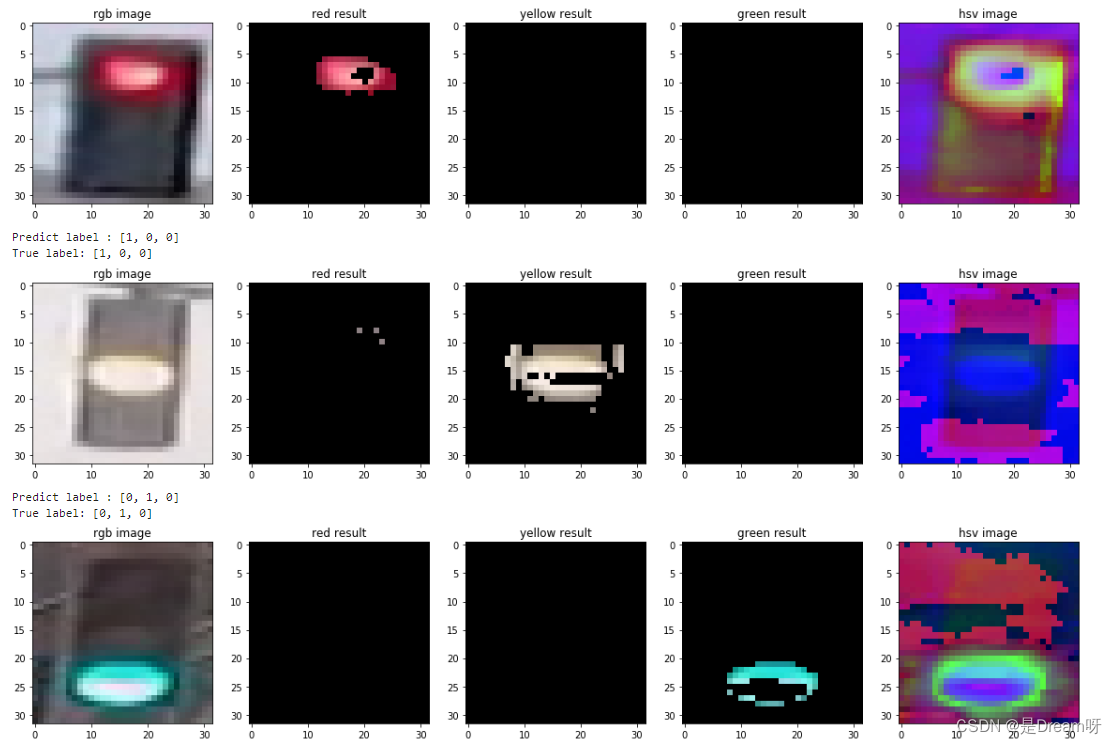## 九、输出准确率

``````def get_misclassified_images(test_images,display=False):
misclassified_images_labels = []
for image in test_images:
# 获取真实数据
im = image
true_label = image
assert (len(true_label)==3),'This true_label is not the excepted length (3).'
#从分类器中获取预测标签
predicted_label = estimate_label(im,display=False)
assert(len(predicted_label)==3),'This predicted_label is not the excepted length (3).'
#比较真实标签和预测标签
if(predicted_label!=true_label):
#如果这些标签不相等，则图像被错误分类
misclassified_images_labels.append((im,predicted_label,true_label))
return misclassified_images_labels
# 查找给定测试集中所有分类错误的图像
MISCLASSIFIED = get_misclassified_images(STANDARDIZED_TEST_LIST,display=False)
#准确率计算
total = len(STANDARDIZED_TEST_LIST)
num_correct = total-len(MISCLASSIFIED)
accuracy = num_correct / total
print('Accuracy:'+str(accuracy))
print('Number of misclassfied images = '+str(len(MISCLASSIFIED))+' out of '+str(total))
``````

Accuracy:0.9797979797979798
Number of misclassfied images = 6 out of 297

### 结论

`本期推荐：`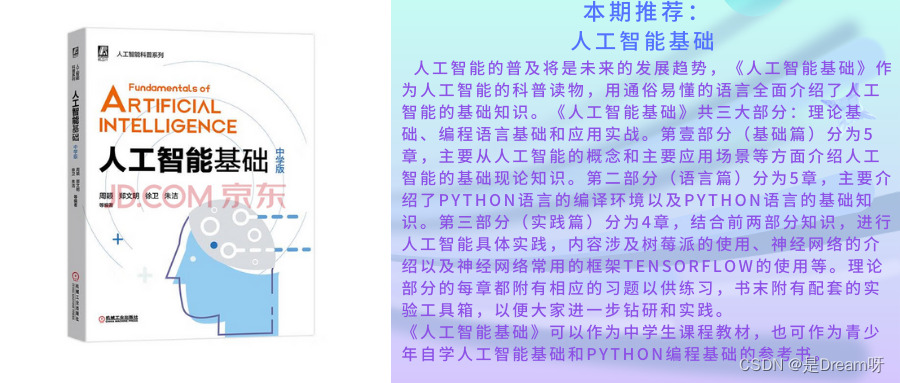`抽奖方式：`评论区随机抽取四位小伙伴免费送出
`参与方式：`关注博主、点赞、收藏、评论区评论“人生苦短，我用Python！”切记要点赞+收藏，否则抽奖无效，每个人最多评论三次！）
`活动截止时间：`2023-06-05 20:00:00